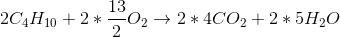# College Chemistry : Identifying Reaction Types

## Example Questions

### Example Question #1 : Identifying Reaction Types

Which of the following is neither a product nor a reactant in a combustion reaction?

Oxygen

All of these

Water

Hydrocarbons

Carbon Dioxide

All of these

Explanation:

A combustion reaction involves the release of heat from the burning of a hydrocarbon in oxygen to form carbon dioxide and water. The amount of hydrogen, carbon, and oxygen are conserved. This reaction is exothermic because of the high energy released when organic hydrocarbons are burned. It can be easily observed in the burning of petroleum distillates such as gasoline.

### Example Question #2 : Identifying Reaction Types

Which type of reaction is shown?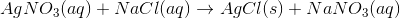Synthesis

Double displacement

Single displacement

Decomposition

Double displacement

Explanation:

There are five main types of chemical reactions.

1) Synthesis reactions have the following format: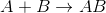In these reactions, two substances combine to form one substance. This occurs when hydrogen and oxygen combine to form water: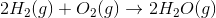2) Decomposition reactions have the following format: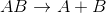In these reactions, a compound breaks down to form smaller substances. This occurs when some acids decompose into an acidic oxide and water: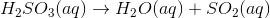3) Single displacement reactions have the following format: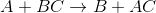In these reactions, a single element replaces another element in a compound. This occurs in the reaction between zinc and hydrochloric acid: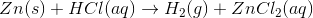4) Double displacement reactions have the following format: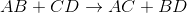In these reactions, an element from each of the two reactant compounds switches places. This occurs in the reaction between sulfuric acid and sodium hydroxide: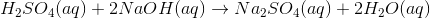5) Combustion reactions have the following format: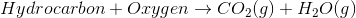In these reactions, a hydrocarbon and oxygen always react to form carbon dioxide and water. Here is an example: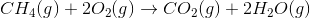### Example Question #3 : Identifying Reaction Types

Identify the type of reaction that is given by the following equation: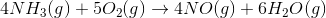Gas evolution

Acid-base

Oxidation-reduction

Precipitation

Oxidation-reduction

Explanation:Recall that combustion equations are examples of reduction-oxidation reactions.

This cannot be a precipitation reaction because there are no solids in the products.

This cannot be a gas-evolution reaction because the reactants are not in aqueous form.

This cannot be an acid-base reaction because there is no proton transfer.

### Example Question #4 : Identifying Reaction Types

What type of reaction is shown?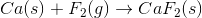Double displacement/metathesis

Single displacement

Combination

Decomposition

Combustion

Combination

Explanation:

This is a basic identify the reaction type equation of the form: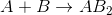Since both reactants are combined into one it is a combination reaction.

Now let's go over the wrong answers:

1) It isn't a single displacement because single displacement reactions are of the form: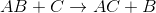2) It isn't a double displacement/metathesis reaction because double displacement reactions are of the form:3) It isn't a decomposition reaction because decomposition reactions are the exact opposite of what occurs in the reaction in the problem. Meaning instead of two or more elements forming a compound, a compound breaks down into two or more elements.4) It isn't a combustion reaction because a combustion reaction refers to the burning of a compound (usually a hydrocarbon) within which the other reactant is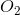and the products are some amount of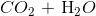. So the general form of a combustion equation look as follows: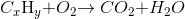### Example Question #7 : Balancing Chemical Equations

What is the balanced chemical equation for the combustion of butane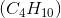?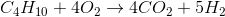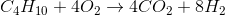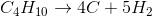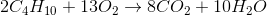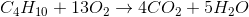Explanation:

Combustion is the chemical reaction of a hydrocarbon with molecular oxygen, and it always produces carbon dioxide and water. Knowing the reactants and products, the unbalanced equation must be: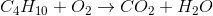We start by balancing the hydrogens. Since there are 10 on the left and only 2 on the right, we put a coefficient of 5 on water.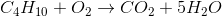Similarly, we balance carbons by putting a 4 on the carbon dioxide.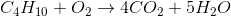To find the number of oxygens on the right, we multiply the 4 coefficient by the 2 subscript on O (which gets us 8 oxygens) and then add the 5 oxygens from the 5 water molecules to get a total of 13. The needed coefficient foron the left would then have to be 13/2.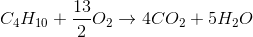Because fractional coefficients are not allowed, we mutiply every coefficient by 2 to find our final reaction: